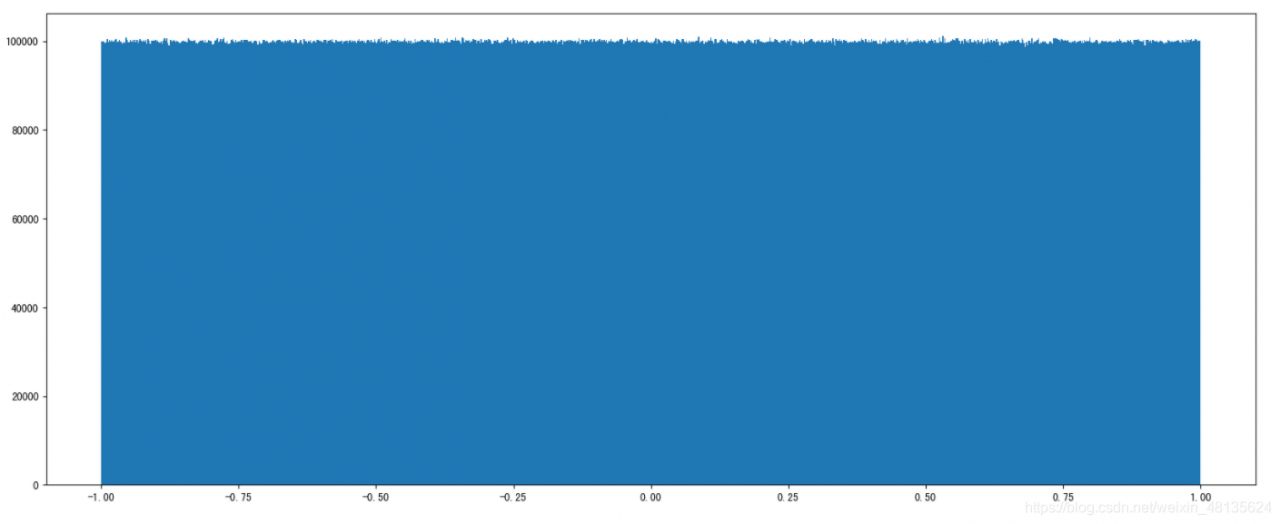# The Usage of Np.random.uniform()

np.random.uniform (low=0.0, high=1.0, size=None)

Function: random sampling from a uniform distribution [low, high]. Note that the definition field is left closed and right open, that is, it contains low but not high

Low: sampling lower bound, float type, default value is 0; high: sampling upper bound, float type, default value is 1; size: output sample number, int or tuple type, for example, size = (m, N, K), then output MNK samples, default value is 1. Return value: ndarray type, whose shape is consistent with the description in the parameter size.

The uniform () method randomly generates the next real number, which is in the range [x, y]

Evenly distributed, left closed, right open

``````np.random.uniform(1.75, 1, 100000000)
#output
array([1.25930467, 1.40160844, 1.53509096, ..., 1.57271193, 1.25317863,
1.62040797])``````

Draw a picture to see the distribution

``````import matplotlib.pyplot as plt
# Generate a uniformly distributed random number
x1 = np.random.uniform(-1, 1, 100000000) # output the number of samples 100000000

# Draw a graph to see the distribution
# 1) Create a canvas
plt.figure(figuresize=(20, 8), dpi=100)

# 2) Plot the histogram
plt.hist(x1, 1000) # x represents the data to be used, bins represents the number of intervals to be divided

# 3) Display the image
plt.show()``````*

*